# NCERT Solutions for Class 11 Chemistry Chapter 12 Organic Chemistry Some Basic Principles and Techniques

NCERT Solutions for Class 11 Chemistry Chapter 12 Organic Chemistry Some Basic Principles and Techniques- In this chapter, students will get to know about some basic principles and techniques of analysis which are required for understanding the properties and formation of organic compounds. The NCERT solutions for Class 11 Chemistry Chapter 12 Organic Chemistry Some Basic Principles and Techniques are prepared by our subject experts to help students in understanding the basic concepts of organic chemistry in a holistic manner. Chapter 12 Organic Chemistry Some Basic Principles and Techniques is an important chapter for a chemistry student from the perspective of getting hold of basic concepts of organic chemistry which will eventually help in subsequent chapters. Since this is a theoretical chapter and many concepts and facts will be learnt from exercise questions directly, hence It is must to go through solutions of NCERT for Class 11 Chemistry Chapter 12 Organic Chemistry Some Basic Principles and Techniques. In this chapter, there are a total of 40 questions in the exercise. You can also refer to NCERT solutions with the detailed explanation of each and every question of NCERT textbook which will help you in preparation of CBSE class 11 final examination as well as in various competitive exams like NEET, JEE Main, BITSAT, VITEEE, etc.

This chapter along with CBSE NCERT solutions for Class 11 Chemistry Chapter 12 Organic Chemistry Some Basic Principles and Techniques discusses some basic concepts in the reactivity and structure of organic compounds which are formed due to the covalent bonding, IUPAC nomenclature and classification of organic compounds, electromeric effect, inductive effect, hyperconjugation, and resonance. All related information about the heterolytic fission and homolytic fission of a covalent bond- carbocations, carbanions, free radicals, nucleophiles, and electrophiles is discussed in the later part of the chapter.

Organic compounds are essential for sustaining life's on the earth and include complex molecules like protein and DNA that constitutes important compounds of our blood, skin, and muscles. Some important areas of application of organic compounds are medicines, dyes, fuels, polymers, and clothing. These compounds can be classified on the basis of their functional group or the structure.

Exercise Questions

What is Functional Group?

A functional group is defined as an atom or group of atoms bonded together in a specific manner, which gives the chemical and physical properties of the organic compounds and they are the centres of the chemical reactivity.

Some important points of Chapter 12 Organic Chemistry Some Basic Principles and Techniques of Class 11 Chemistry-

1. Organic compounds are formed due to covalent bonding.

2. According to orbital hybridization concept carbon can have and hybridized orbitals.

3. The 3D representation of organic compounds on paper can be drawn by dash and wedge formula.

4. Compounds having the same molecular formula but differ in their physical and chemical properties are known as isomers and the phenomenon is called isomerism.

5. Addition, substitution, elimination and rearrangement reactions are the types of organic reaction.

## Topics of NCERT Grade 11 Chemistry Chapter 12 Organic Chemistry Some Basic Principles and Techniques

12.1 General Introduction

12.2 Tetravalence of Carbon: Shapes of Organic Compounds

12.3 Structural Representations of Organic Compounds

12.4 Classification of Organic Compounds

12.5 Nomenclature of Organic Compounds

12.6 Isomerism

12.7 Fundamental Concepts in Organic Reaction Mechanism

12.8 Methods of Purification of Organic Compounds

12.9 Qualitative Analysis  of Organic Compounds

12.10 Quantitative Analysis

## Exercise Questions

c1              c2

Here  carbon is  hybridised and the  carbon is  hybridised.

### Q 12.1(ii)     What are hybridisation states of each carbon atom in the following compounds ?

c1       c2              c3

Here  caron is  hybridised,  carbon is  and the third carbon atom is  hybridise

Here is the structureHere  and  carbon atom is in  hybridistaion and the  carbon is

In benzene, all the six carbon atoms are  hybridised.

1               2             3

Here, in the above compound the  carbon atom is hybridised,  carbon atom is hybridised and the  carbon is  hybridiesd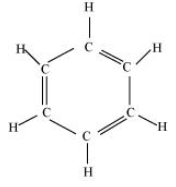There are six C-C sigma bonds, six C-H sigma bonds and three -bonds between the carbon atom in the benzene.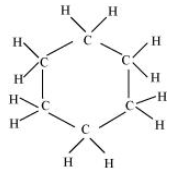In , there are six sigma bond between C-Cand twelve sigma bond between C-H bonds in the given compound.

In ,There are two sigma C-H bonds and two C-Cl sigma bonds.In this compound, there are two C-C sigma bonds, four C-H sigma bonds and two  bonds between carbon atoms.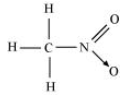There are three C-H sigma bonds, one C-N sigma bond, one N-O sigma bond and one N-O -bonds.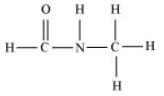Two C-N sigma bonds, four C-H sigma bonds, one N-H sigma bonds and one  -bond in the above compound.

Bond line formula of following compounds-

 Isopropyl alcohol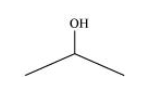2, 3-Dimethyl butanal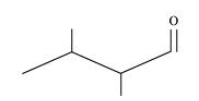Heptan-4-one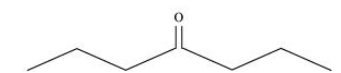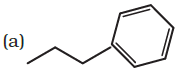The IUPAC name of the above compound is 1-phenylpropane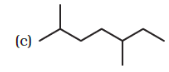The IUPAC name of the above structure is 2, 5-dimethyl heptane.

The IUPAC name of the compound is 1, 1-dichloro-2-ethanol

(a) 2,2-Dimethylpentane or 2-Dimethylpentane

(b) 2,4,7- Trimethyloctane or 2,5,7-Trimethyloctane

(c) 2-Chloro-4-methylpentane or 4-Chloro-2-methylpentane

(a) The prefix  in the IUPAC nomenclature is indicating two identical substituent group in the parent chain. Since two methyl group present at  of the parent chain. So, the correct IUPAC name is 2, 2-dimethylpentane.

(b) In IUPAC nomenclature the sum of locant number should be minimum. Here the sum of 2,4,7 (=13)is less than the sum of 2, 5, 7 (=14). Thus the correct IUPAC name is 2,4,7- Trimethyloctane

(c) In IUPAC nomenclature, if the substituent group acquires the equivalent position of the parent chain then the lower number is given to one that comes first in alphabetical order. Hence 2-Chloro-4-methyl pentane is the correct IUPAC name of the compound.

(d) If the two functional groups are present in the parent chain then the suffix of the IUPAC name depends on the principal functional group. Here alcohol is the principal function group so the suffix should be  and alkyne group considered as a substituent group. Therefore the correct IUPAC name of the compound is But-3-yn-1-ol.

the first five members of each homologous series beginning with the following compound are shown as-

---methanoic acid

• ethanoic acid

• propanoic acid

• butanoic acid

• pentanoic acid

the first five members of each homologous series beginning with the following compound are shown as-

(propanone)

• Butanone

• Pentan-2-one

• hexan-2-one

• Hept-2-one

The first five members of each homologous series beginning with the following compound are shown as-
..........(Ethene)

• Propene

• 1-Butene

• 1-Pentene

• 1-Hexene

### Q 12.7(a)     Give condensed and bond line structural formulas and identify the functional group(s) present, if any, for :                 2,2,4-Trimethylpentane

2,2,4-Trimethylpentane

Condensed formula-

Bond line formula-The condensed formula of 2-Hydroxy-1,2,3-propanetricarboxylic acid

The bond line structure -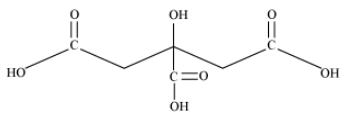the condensed formula of hexanedial

The bond line structure is-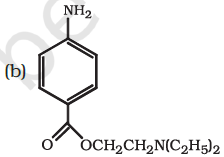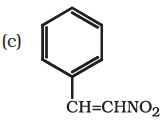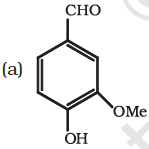The functional groups in the above structure are-

1. Aldehyde ()

2. HYdroxyl ()

3. Methoxy ()

4. double bond

(b)The following functional groups are presents-

1. Amino, primary amine ()

2. Ester ()

3. Tertiary amine ()   R = ethyl groupHere,

1. Nitro group ()

2. double bond

Since Nitro group is an electron withdrawing group. So, it shows  effect, By reducing the electron negative charge of the compound, it stabilises the compound. On the other hand, the methyl group is an electron donor group so it shows  effect. This increases the negative charge on the compound and destabilises the compound.

Hence    is more stable than the .

When an alkyl group is attached to the  system, it acts as an electron donor group by the property of hyperconjugation.
for example in propene,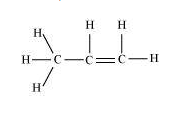In figure, you can see that the sigma electrons of C-H bonds of the alkyl group are delocalised because of the partially overlapping of the  sigma bond orbital with the empty p orbital of the  bond of the adjacent carbon atom. It is also known as no-bond resonance.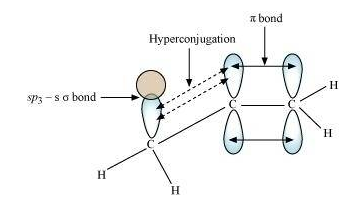The resonating structure of the phenol-
The lone pair of electron starts shifting to Oxygen - carbon bond and form a double bond character and  electron of C-C bond shift to next C-C single bond.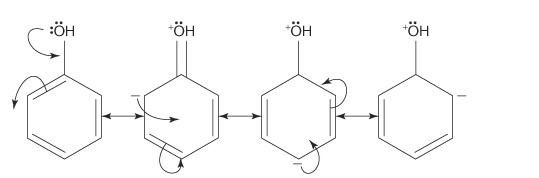The resonating structure of the nitrobenzene-

Here the electrons of N-O bonds shifts to Oxygen atom (more electronegative) and then the  electron of carbon-carbon double bonds starts delocalising towards the N atom.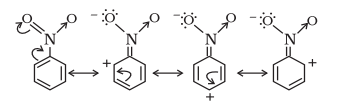The resonating structure of the But-2-ene-1-al-
Here the electrons of C-O bonds shifts to Oxygen atom (more electronegative) and then the  electron of carbon-carbon double bonds starts shifting towards the next C-Cbond, introducing a partial double bond character.The resonating structure of the Benzaldehyde-
Here the  electron of carbon-oxygen double bond starts shifting towards the electronegative oxygen atom and the -electrons of C-C double bonds shifting towards carbonyl group (as shown in figures).The resonating structure of Benzyl carbocation-
The -electrons of C-C double bond shift towards the  bond to minimise the deficiency of electron density and the rest of the -electrons follow the same process.The resonating structure of But-2-ene-1-yl carbocation-
The -electrons of C-C double bond shift towards the  bond to minimise the deficiency of electron density at . So, this way only one resonant is possible.Electrophile- It is an electron deficient species, which seeking for an electron pair. This reagent takes away an electron pair. It is denoted as . For example, carbocationised  neutral molecule having functional groups such as carbonyl group are an example of the electrophile.

A nucleophile is a reagent that brings an electron pair. In other words, it is nucleus- seeking reagent called nucleophile.
For examples-  and carbanions () etc. Ammonia and water also act as a nucleophile due to the presence of lone pair of electron.

Electrophiles are electron deficient species and can be seeking for an electron pair. On the other hand, the nucleophile is electron rich reagents and they can electron donor(nucleus seeking).

Therefore,
(a)  have one lone pair of an electron, electron rich species. So, it is a nucleophile

(b)  acts as a nucleus seeking reagent and act as a nucleophile

(c)  , it is an electron deficient species, So, it is electrophile.

It is a substitution reaction as in this above equation the bromine group is replaced by the  group.

The given reaction is an example of an addition reaction because in this reaction the two reactant molecule combined to form a single product.  Also, we can say that the Hydrogen and chlorine of  is added in the two different carbon atom of the same compound.

In this reaction, the hydrogen and bromine are removed from the original compound and formed ethene. So, this is an elimination reaction.

In the above reaction, the substitution takes place between the two reactants, followed by the rearrangement of atoms and the groups of atoms.

### Q 12.15     What is the relationship between the members of following pairs of structures ? Are they structural or geometrical isomers or resonance contributors ?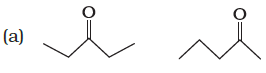These are pairs are structural isomers.
Compounds having the same molecular formula but different structures are called structural isomers. The above compounds have the same molecular formula but the structures are different due to the difference in the position of the carbonyl group.
In structure one -CO group is present at  position and in second the -CO group is present at   position.

(a)Bond cleavage by using curved-arrows to show the electron flow of the given reaction can be represented asIt is an example of homolysis because the two electrons are equally divided into the products.(see in fig)

### Q 12.16(b)     For the following bond cleavages, use curved-arrows to show the electron flow and classify each as homolysis or heterolysis. Identify reactive intermediate produced as free radical, carbocation and carbanion.

Bond cleavage by using curved-arrows to show the electron flow of the given reaction can be represented as;It is an example of heterolytic cleavage as the bond breaks in such a manner that electron pair will remain with the carbon of propanone. The reaction intermediate is carbanion.Bond cleavage by using curved-arrows to show the electron flow of the given reaction can be represented as;It is heterolysis as shared pair of an electron is distributed to only bromine ion. Here the reaction intermediate is carbocation.Bond cleavage by using curved-arrows to show the electron flow of the given reaction can be represented asThe reaction intermediate is carbocation. It is a heterolytic cleavage as the bonds break in such a manner that shared&nbnbsp;electron pair will remain with the one species.

Inductive effect- The permanent displacement of sigma () electrons along the saturated chain, whenever an electron donating or withdrawing group is present, is known as the inductive effect. It could be effect.

Electromeric effect- The complete transfer of the shared pair of -electrons to one of the atoms linked by multiple bonds on the demand of attacking reagent. It is a temporary effect. It may be +E(electrons transferred towards attacking reagent) or -E effect(electron transferring away from the attacking reagent).

(a) The order of acidity can be explained by the negative inductive effect (). As the no. of chlorine atom increases, the  effect also increases and so, the acidic strength also increases.

(b) This can be explained by the  effect of the alkyl group. As the number of electron donor group increases,  effect will also increase. With the increases in  effect, the acidic character decreases accordingly.

Crystallisation

Crystallisation-
It is one of the most commonly used techniques for the purification of solid organic compounds. Its principle is based on the difference in the solubilities of the compounds and the solvent's impurities. The impure compounds are dissolved in solvent but they are sparingly soluble at room temperature but soluble at the higher temperature. On cooling the compound, the pure compounds get crystallise and removed by filtration.
For example- pure aspirin is obtained by recrystallising crude aspirin. Around 2 - 4 g of crude aspirin is dissolved in 20 mL of ethyl alcohol and the solution is heated for complete dissolution. Then after crystal formation, they can filter out and dried.

Distillation

Distillation-
This method is used for the purification of liquids from non-volatile impurities. It is based on the fact that fluids of having different boiling points vaporise at different temperatures. The impure liquid is boiled in a flask, and initially, the vapours of lower boiling points component are formed. The vapours are condensed by using a condenser, and the liquid is collected in a receiver.
For example- Organic liquids such as benzene, toluene, xylene etc can be purified by this method.

Chromatography

Chromatography-
It is one of the extensive methods for the separation and purification of organic compounds. It is based on the difference in the movements of components of mixtures through the stationary phase under the influence of the mobile phase. The stationary phase can be solid or liquid. While the mobile phase is only liquid or gas.
For example- This technique can separate a mixture of blue and red ink.

Fractional distillation can be used to separate the two compounds with different solubilities in a solvent S. The following steps are carried out in this process-

1. The powdered mixture is taken in a flask, and the solvent is added into it and stirred simultaneously. We have to prepare a saturated solution and then heat it.

2. After heating, this hot saturated solution can be filtered with filter paper in a china dish.

3. Now the solution is allowed to cool. The less soluble compounds crystalise first, and more soluble compounds remain in the solution. After removing these crystals, the latter is concentrated once again. The hot solution is allowed to cool, and then the crystals of the more soluble compound are obtained.

4. The last step is isolation and drying of crystals from the mother liquor.

The difference between distillation, distillation under reduced pressure and steam distillation are-

 Distillation Distillation under reduced pressure Steam Distillation 1. Used for the purification of the compounds that are non-volatile impurities or liquids, which don't decompose on boiling. 1. Used to purify liquids, which tends to decompose on boiling. Under the condition of reduced pressure. 1.Used to purify the organic compounds, which is steam volatile and immiscible with water. SImply, to separate the volatile liquids from non-volatile impurities. Or a mixture of liquids having sufficient Boiling point difference. The liquid will boil at low temperature than its boiling points, therefore, it does not decompose. The mixture of water and aniline can be separated by this method. The mixture of petrol and kerosene is separated by this method. Glycerol is purified by this method.

Lassaigne’s test.-
This test is used to detect the presence of nitrogen, sulphur, halogens and sulphur in organic compounds. These elements are present in the covalent form in an organic compound. So, they are converted into the ionic form by fusing the compound with the sodium metal.
(X = Cl, Br, I)

Cyanide, sulphide or halide of sodium are extracted from the fused mass by boiling it with distilled water. This Is known as sodium extract method or Lassaigne's extract.

Dumas method

In the Dumas method, Nitrogen containing organic compound is heated with the copper oxide in carbon dioxide atmosphere, yields free nitrogen in addition to  and water.

Traces of nitrogen oxides can also be formed in the reaction, which can be reduced to nitrogen by passing the gaseous mixture over the heated copper gauge. The produced mixture of gases is collected over by an aqueous solution of , it absorbs . Nitrogen is collected in the upper part of the graduated tube.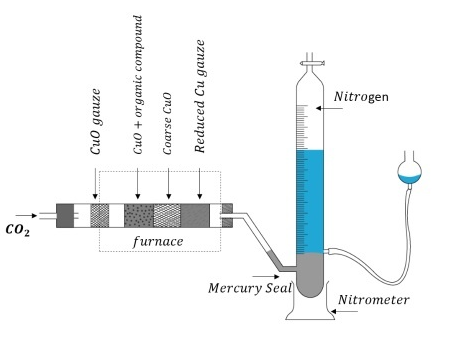Kjeldahl’s method.

In Kjeldahl’s method, the nitrogen-containing organic compound is heated with concentrated sulphuric acid. Nitrogen is converted into ammonium sulphate. It is then passed into the known volume of sulphuric acid. The amount of ammonia produced can be estimated by the amount of  consumed in the reaction.

It is done by estimating the amount of unreacted  left after the absorption of ammonia by titrating it with a standard alkali solution. This method is not applicable for the compound contain nitrogen in -nitro form or nitrogen present in the ring structure.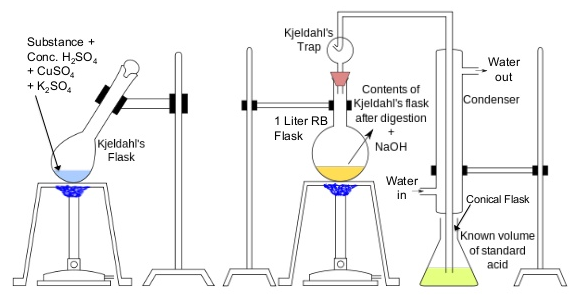Estimation of halogen is done by Carius method. In this method, a known quantity of organic compound is heated with fuming  (nitric acid) with the presence of silver nitrate, contained in a hard glass tube, known as carius tube. C and H present in the compound are oxidised to carbon dioxide and water. And halogens are into to form  and then it is filtered, dried, and weighed.

Let the mass of the organic compound be  gram.
Mass of  formed =  gram
1 mol of  contains 1 mol of X.
Therefore, Mass of halogen inm1 g of AgX = (Atomic mass of ) (Molecular mass of )

Thus, % of halogen will be  =   (Atomic mass of  mol. wt of

Estimation of sulphur- In this method, Organic compound is heated either fuming nitric acid or sodium peroxide in a hard glass tube called carius tube. Sulphur present in the compound is oxidised to form sulphuric acid. It is precipitated by as barium sulphate by adding barium chloride solution in water. Then ppt is filtered, washed and weighed.

Let the mass of organic compound taken =   g
and the mass of barium sulphate formed =  g

1 mol of  = 233 g  = 32 g sulphur

g   contains = g sulphur
Percentage (%)of sulphur =

Estimation of phosphorus-  In this process, a known mass of an organic compound is heated with fuming nitric acid and the phosphorus gets oxidised to phosphoric acid. By adding ammonia and ammonium molybdate, phosphorus can be precipitated as ammonium phosphomolybdate, . It can be also estimated as by precipitating it as  by adding magnesia mixture which on ignition yields .

Let the mass of organic compound taken =  g and mass of ammonium phosphomolybdate =  g

Molar mass of  g
Percentage(%) of phosphorus =

If phosphorus is estimated as ,
Percentage(%) of phosphorus = %

In paper chromatography, chromatography paper is used. It contains water trapped in it, which acts as the stationary phase. The solution of the mixture is spotted on this base of chromatography paper. The strip of paper is suspended in a suitable solvent, which is the mobile phase. Due to capillary action, the solvent rises up in the paper and it flows over the spot. The spots of the different component travel with the mobile phase to a different level of heights. The obtained paper is called chromatogram.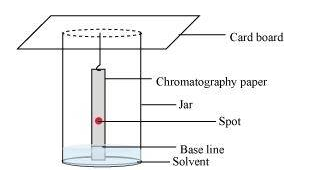Nitric acid added to sodium extract before adding silver nitrate for testing halogens to decompose the   to  and  to  and to expel these gases. If any ampount of nitrogen and sulphur are present in the form of  and , then they are removed.

In Organic compounds, nitrogen, sulphur and halogens are covalently bonded. To detect them, they have to convert into ionic form. This is done by the fusing of an organic compound by the sodium metal. This is known as Lassaigne's test.
The following chemical reactions are-

Here X = halogen atoms

To separate a mixture of calcium sulphate and camphor, we use sublimation method. In this method, the sublimable compound is converted to vapour state from the solid state without achieving its liquid states. Here camphor is sublimable compound and calcium sulphate is not.

In steam distillation, Organic liquid starts boiling when the total sum of the vapour pressure of an organic liquid (P') and the of water (P'') becomes equal to the atmospheric pressure (P),
it means P = P' + P''. since P'< P'', the orgnic liquid will vaporise at a lower temperature than its boiling point.

No,  does not give white ppt of  on heating with the silver nitrate. This is because the chlorine atoms are covalently bonded with the carbon atom. To get ppt, it should be present in ionic form.

Carbon dioxide is acidic in nature and  is a strong base. Thus, carbon dioxide reacts with the potassium hydroxide by acid-base reaction and form a salt known as potassium carbonate and water as a by-product.

Thus, due to the increase in KOH the mass of U-tube is also increases. This increased mass of the tube gives the mass of carbon dioxide produced. From its mass, the % of carbon in the Organic compound can be estimated.

It is necessary to use acetic acid and not sulphuric acid for acidification of sodium extract for testing sulphur by lead acetate test because in case of sulphuric acid the complete ppt formation of lead sulphate does not take place. While the addition of acetic acid will ensure a complete ppt. formation of sulphur in the form of lead sulphate due to the common ion effect.

Given,
Percentage of carbon = 69%
% of Hydrogen = 4.8%, and
% of Oxygen  =  26.2 %

So, 0.2 g of OC have g of Carbon = 0.138g
we know that,
molecular weight of   = 44 g
12 g of C is present in 44 g of

Therefore, 0.138 g of C present in  = 0.506 g of

Hence, from 0.2 g of OC 0.506 g of  will be produced.

Similarly,
100 g  of OC contains 4.8 g of H
So, 0.2 g of OC contains  = 0.0096 g of H
We know the mol. wt. of water = 18 g

Therefore 0.0096 g of H will present in  = 0.0864 g of water

Hence 0.2 gram of OC will produce 0.0864 g of water on complete combustion of

Given that,
total mass of organic compound = 0.50 g
60 mL of 0.5 M solution of   required for neutralisation by residual acid.

60 mL of 0.5 M   solution =   mL of 0.5M   = 30 mL of 0.5 M

Therefore,
Acid consumed in absorption of evolved ammonia is (50-30) mL = 20 mL

Again, 20 mL of 0.5 M  = 40 mL of 0.5 M

Also, since 1000 mL of 1 M  contains 14 g of nitrogen,
So,  40 mL of 0.5 M   will contain =  g of Nitrogen

Therefore, percentage(%) of nitrogen in 0.50 g of organic compound  =  = 56 %

Given that,
Mass of organic compound = 0.3780 g.
Mass of AgCl formed = 0.5740 g

It is known that,
1 mol of  contains 1 mol of Cl.

Thus, mass of chlorine in 0.5740 g of

= 0.1421 g

∴ Percentage(%) of chlorine =   = 37.59%

Hence, the percentage of chlorine present in the given organic compound is 37.59%.

Given data,

Total mass of the OC = 0.468 g
Mass of barium sulphate formed = 0.668 g

We know that,

1 mol of BaSO4 = 233 g of  = 32 g of sulphur
Thus, 0.668 g of  contains =  g of sulphur = 0.0917 g of sulphur

Therefore, the percentage(%) of sulphur

= 19.59 %

Hence, the percentage of sulphur in the given compound is 19.59 %.

&nbnbsp;

In the organic compounds-

1               2             3               4         5

In  and  carbon atom the hybridisation is
So, the correct option is (c)

The Prussian blue colour is due to the formation of   in Lassaigne's test.

So, the correct option is (b)

### Q 12.38.    Which of the following carbocation is most stable ?

We know the stability of carbocation order is-
(It is due to the tendency of the methyl group to electron release and stabilise the carbocation)

So, the correct option is (b)

Chromatography is the latest technique of separation and purification of organic compounds.
So, the correct option is (d)

(a) electrophilic substitution         (b) nucleophilic substitution      (c) elimination        (d) addition

Here,  attacks on ethyl iodide and substitute the iodide ion ion.  having a lone pair of an electron, so it acts as a nucleophile. Hence it is a nucleophilic substitution reaction.
So, the correct option is (b)

## NCERT solutions for class 11 chemistry

 Chapter 1 NCERT solutions for class 11 chemistry chapter 1 Some Basic Concepts of Chemistry Chapter-2 CBSE NCERT solutions for class 11 chemistry chapter 2 Structure of Atom Chapter-3 Solutions of NCERT class 11 chemistry chapter 3 Classification of Elements and Periodicity in Properties Chapter-4 NCERT solutions for class 11 chemistry chapter 4 Chemical Bonding and Molecular Structure Chapter-5 CBSE NCERT solutions for class 11 chemistry chapter 5 States of Matter Chapter-6 Solutions of NCERT class 11 chemistry chapter 6 Thermodynamics Chapter-7 NCERT solutions for class 11 chemistry chapter 7 Equilibrium Chapter-8 CBSE NCERT solutions for class 11 chemistry chapter 8 Redox Reaction Chapter-9 Solutions of NCERT class 11 chemistry chapter 9 Hydrogen Chapter-10 NCERT solutions for class 11 chemistry chapter 10 The S-Block Elements Chapter-11 CBSE NCERT solutions for class 11 chemistry chapter 11 The P-Block Elements Chapter-12 NCERT solutions for class 11 chemistry chapter 12 Organic chemistry some basic principles and techniques Chapter-13 NCERT solutions for class 11 chemistry chapter 13 Hydrocarbons Chapter-14 CBSE NCERT solutions for class 11 chemistry chapter 14 Environmental Chemistry

## NCERT Solutions for Class 11- Subject wise

 NCERT solutions for Class 11 Biology NCERT solutions for Class 11 Maths NCERT solutions for Class 11 Chemistry NCERT solutions for Class 11 Physics

## Benefits of NCERT Solutions for Class 11 Chemistry Chapter 12 Organic Chemistry Some Basic Principles and Techniques?

• Solutions for NCERT Solutions for Class 11 Chemistry Chapter 12 Organic Chemistry Some Basic Principles and Techniques has been created in such a way you can learn and remember easily.
• The solutions are provided here are as per CBSE guidelines.
• Along with NCERT solutions for chapter 12, you will get other chapter solutions as well.
• Through NCERT Solutions for Class 11 Chemistry Chapter 12 Organic Chemistry Some Basic Principles and Techniques, why do we fall ill, you will get in-depth knowledge of all the concepts which will help you to score well in your exams.